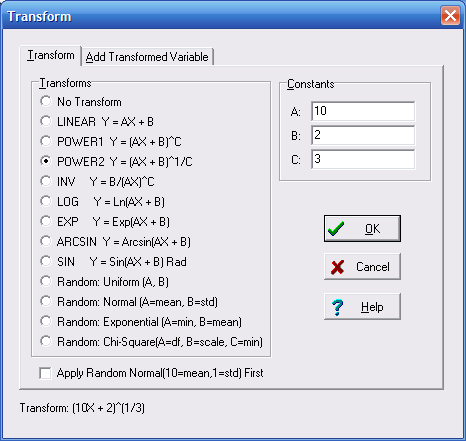# Transforming Data

The Data Editor has the ability to enter a transform equation. When a transform equation is active the data will be transformed during analysis. In addition, a transformed column may also be generated and added to the Data Editor. (See Add Transform Variable.)

## To Specify a Transform Equation for Analysis

1. Select Data|Transform from the menu.
2. The following form will be displayed.3. Select the Transform Equation.
4. Enter the Constant for the Equation.
5. Click the OK Button

## To Turn Off the Transform

Select No Transform for the Transform equation.